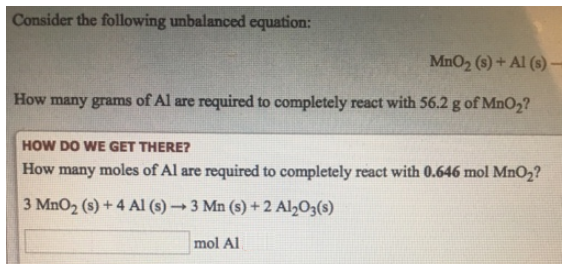# Problem: Consider the following imbalanced equation: MnO2 (s) + Al (s) →How many grams of Al are required to completely react with 56.2 g of MnO2? How many moles of Al are required to completely react with 0.646 mol MnO2? 3MnO2 (s) + 4Al (s) → 3Mn (s) + 2Al2O3 (s)

###### FREE Expert Solution
88% (37 ratings)###### Problem Details

Consider the following imbalanced equation:

MnO2 (s) + Al (s) →

How many grams of Al are required to completely react with 56.2 g of MnO2

How many moles of Al are required to completely react with 0.646 mol MnO2

3MnO2 (s) + 4Al (s) → 3Mn (s) + 2Al2O3 (s)